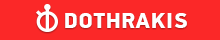GMAT Question of the Day - Daily to your Mailbox; hard ones only

 It is currently 26 Jun 2019, 03:18GMAT Club Daily Prep

Thank you for using the timer - this advanced tool can estimate your performance and suggest more practice questions. We have subscribed you to Daily Prep Questions via email.

Customized
for You

we will pick new questions that match your level based on your Timer History

Track

every week, we’ll send you an estimated GMAT score based on your performance

Practice
Pays

we will pick new questions that match your level based on your Timer History

Not interested in getting valuable practice questions and articles delivered to your email? No problem, unsubscribe here.In the xy-plane, line k and line p intersect at 90-degree angles. If t

Author Message
TAGS:

Hide Tags

Math ExpertV
Joined: 02 Sep 2009
Posts: 55802
In the xy-plane, line k and line p intersect at 90-degree angles. If t  [#permalink]

Show Tags00:00

Difficulty:

(N/A)

Question Stats:71% (00:22) correct29% (01:10) wrongbased on 39 sessions

HideShow timer StatisticsIn the xy-plane, line k and line p intersect at 90-degree angles. If the slope of line k is 1/2, what is the slope of line p ?

A. -2
B. −1/2
C. 0
D. 1/2
E. 2

_________________
CEOP
Joined: 18 Aug 2017
Posts: 3935
Location: India
Concentration: Sustainability, Marketing
GPA: 4
WE: Marketing (Energy and Utilities)
Re: In the xy-plane, line k and line p intersect at 90-degree angles. If t  [#permalink]

Show Tags

Bunuel wrote:
In the xy-plane, line k and line p intersect at 90-degree angles. If the slope of line k is 1/2, what is the slope of line p ?

A. -2
B. −1/2
C. 0
D. 1/2
E. 2

for two lines which are perpendicular to each other i.e. intersect at 90 degree product of their slopes has to be equal to -1

so m1*1/2= -1
m1= -2 IMO A
_________________
If you liked my solution then please give Kudos. Kudos encourage active discussions.
VPD
Joined: 09 Mar 2016
Posts: 1274
Re: In the xy-plane, line k and line p intersect at 90-degree angles. If t  [#permalink]

Show Tags

Bunuel wrote:
In the xy-plane, line k and line p intersect at 90-degree angles. If the slope of line k is 1/2, what is the slope of line p ?

A. -2
B. −1/2
C. 0
D. 1/2
E. 2

the slopes of perpendecular lines are negative reciprocals of each other: m1*m2 = -1

So, $$m1*\frac{1}{2} =-1$$

$$M1 = -2$$Re: In the xy-plane, line k and line p intersect at 90-degree angles. If t   [#permalink] 01 Dec 2018, 04:39
Display posts from previous: Sort by

In the xy-plane, line k and line p intersect at 90-degree angles. If t